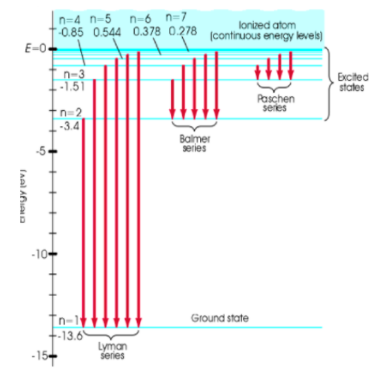Problem: Determine the frequency of the fourth Lyman line (n = 5 to n = 1transition) using the figure below.  What photon energy does that correspond to?

FREE Expert Solution
87% (316 ratings)
Problem Details

Determine the frequency of the fourth Lyman line (n = 5 to n = 1transition) using the figure below.  What photon energy does that correspond to?